Solutions to the SIAM 100-Dollar, 100-Digit Challenge

Team: Gaston Gonnet, Informatik, ETH, Zurich, and Robert Israel, Mathematics, UBC.
Most of these solutions are the ones that actually produced the results we sent in, but I (R.I.) have changed a few of them. All these solutions use Maple.

Each problem had an answer that was a single real number, of which 10 correct digits were required.

The complete results are at http://www.comlab.ox.ac.uk/oucl/work/nick.trefethen/hundred.html. Our team was one of 20 first prize winners. See our prize.

Problem 1:

What is?

Solution

Problem 2:

A photon moving at speed 1 in the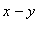plane starts at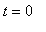atheading due east.  Around every integer lattice pointin the plane, a circular mirror of radiushas been erected.  How far from the origin is the photon at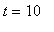?

Problem 3:

The infinite matrixwith entries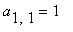,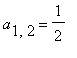,,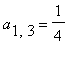,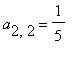,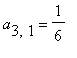, etc., is a bounded operator on.  What is ||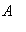||?.

Problem 4:

What is the global minimum of the function?

Solution

Problem 5:

Let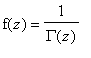, whereis the gamma function, and let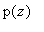be the cubic polynomial that best approximateson the unit disk in the supremum norm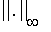.  What is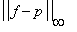?

Problem 6:

A flea starts at (0,0) on the infinite 2D integer lattice and executes a biased random walk: At each step it hops north or south with probability 1/4, east with probability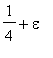, and west with probability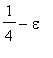.  The probability that the flea returns to (0,0) sometime during its wanderings is 1/2.  What is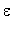?

Problem 7:

Letbe the 20,000 x 20,000 matrix whose entries are zero everywhere except for the primes 2, 3, 5, 7, ..., 224737 along the main diagonal and the number 1 in all the positions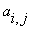with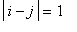,2,4,8,...,16384.  What is the (1,1) entry of?

Problem 8:

A square plate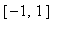xis at temperature.  At timethe temperature is increased to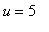along one of the four sides while being held at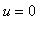along the other three sides, and heat then flows into the plate according to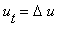.  When does the temperature reachat the center of the plate?

Problem 9:

The integral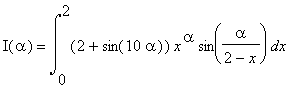depends on the parameter.  What is the value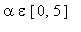at which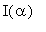achieves its maximum?

Problem 10:

A particle at the center of a 10 x 1 rectangle undergoes Brownian motion (i.e. 2D random walk with infinitesimal step lengths) till it hits the boundary.  What is the probability that it hits at one of the ends rather than at one of the sides?